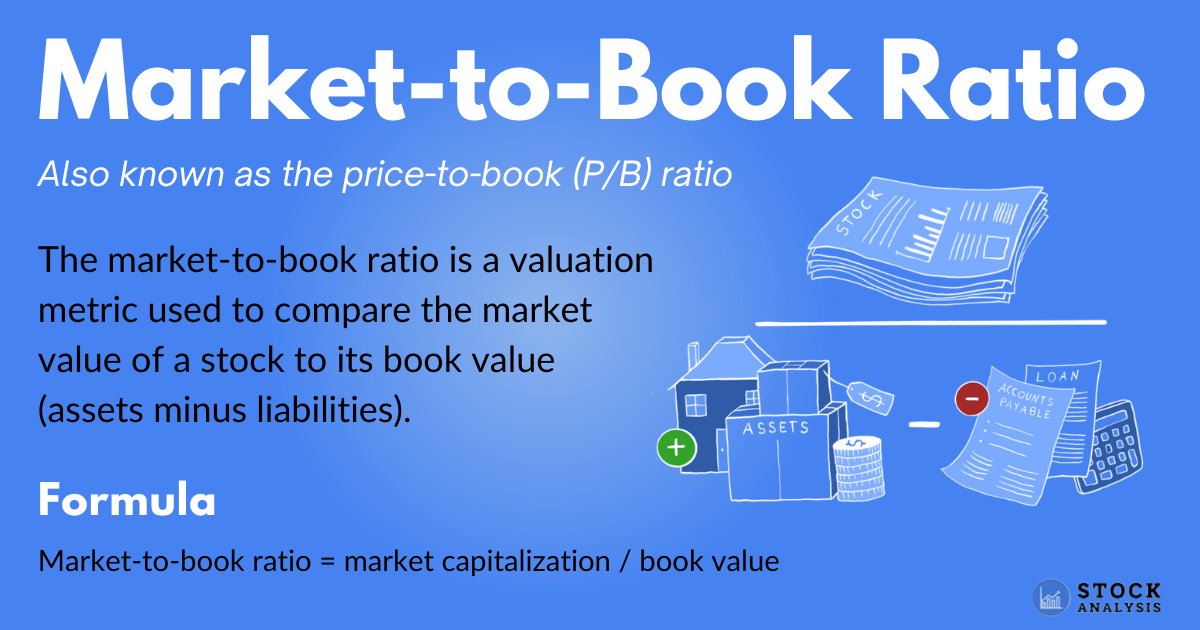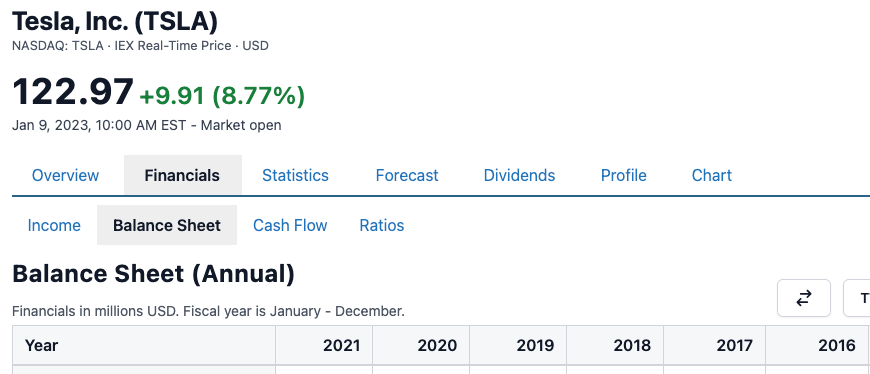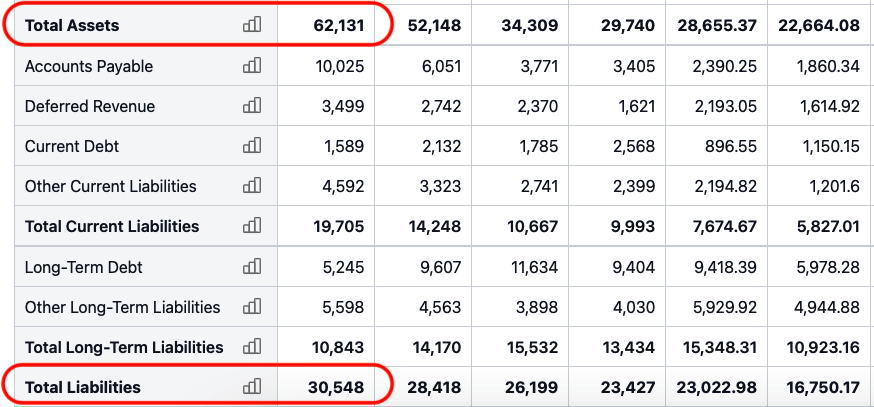# Market-to-Book Ratio

The market-to-book ratio is a valuation metric used to compare the market value of a stock to its book value.

It's calculated by dividing a company's market cap by its book value, like so:

Market-to-book ratio = market capitalization / book value

Investors can use the market-to-book ratio to determine whether a stock is over or undervalued. A high ratio indicates overvaluation, while a low ratio indicates undervaluation.

The market-to-book ratio is also called the price-to-book (P/B) ratio.

Below is an overview, including how to use the market-to-book ratio when evaluating stocks.

## What is the market-to-book ratio?The market-to-book ratio is a metric that assesses whether a stock is over or undervalued.

It's calculated by dividing the market cap by the book value, showing you how the market value compares to the value as listed on financial documents.

• Market cap: Short for market capitalization, this is the value of a company's shares of stock. It's determined by multiplying the stock price by the number of shares outstanding.
• Book value: This is the net value of a company's assets, or book equity value. It's determined by subtracting liabilities from assets, resulting in the theoretical amount of money left if all the assets were sold and all the liabilities were paid.

A high market-to-book ratio indicates that a stock is expensive, or overvalued, while a low ratio indicates that it is cheap, or undervalued.

So-called value stocks often have a low market-to-book ratio, which indicates that you can buy the stock for a low price relative to the value of its assets.

Summary

The market-to-book ratio is a valuation metric used to assess whether shares of stock are over or undervalued. It does so by comparing a company's market value to its book price.

## How to calculate the market-to-book ratio

The market-to-book ratio is calculated by dividing a company's market cap by its book value:

Market-to-book ratio = market capitalization / book value

You can either calculate this manually or use sites like Stock Analysis. Either way, it's helpful to understand how the components of the market-to-book ratio are calculated.

To calculate market cap, simply multiply the stock price by the number of shares outstanding:

Market cap = stock price x total shares outstanding

The simplest way to calculate book value is by subtracting all liabilities from all assets, like so:

Book value = total assets - total liabilities

You can find total assets and liabilities listed on a company's balance sheet. The book value may also be shown on the balance sheet, under shareholders' equity.

It's worth noting that some sources use slightly different formulas to calculate book value.

Additionally, you can also calculate the market-to-book ratio by dividing the stock price by the book value per share.

Market-to-book ratio = stock price / book value per share

Summary

The market-to-book ratio is a simple calculation that divides market cap by book value. You can either calculate it yourself using balance sheet inputs or use financial data websites.

## Example market-to-book ratio calculation

Let's calculate the market-to-book ratio for a real company.

At the beginning of 2022, Tesla (TSLA) stock was trading for \$120.94 per share, with a market cap of USD \$406 billion.

For 2021, the company's balance sheet listed its total assets, in millions, at \$62,131, and its total liabilities at \$30,548.Source: Tesla's Balance Sheet

The market-to-book ratio can then be calculated in two steps:

Book value: \$62,131 - \$30,548 = \$31,583 (in millions)

Market-to-book ratio: 406,000 / 31,583 = 12.85

In other words, you are paying \$12.85 dollars for each dollar of net assets.

Summary

Tesla's market-to-book ratio in early 2022 was 12.85, meaning you'd pay \$12.85 for each dollar of net assets. This shows you the price of the stock relative to the book price.

## How to use the market-to-book ratio

The market-to-book ratio, or price-to-book ratio, is one of the most commonly used ratios to determine whether a company's stock is under or overvalued.

At a high level, a ratio below one may indicate that a stock is very cheap, while a high ratio, such as over three, may suggest that it is expensive.

However, these can be arbitrary numbers, as a company's type, age, and other factors can affect the ratio. For this reason, it's best to compare one company's ratio to that of a peer in the same industry and same stage of growth.

Moreover, when companies are trading for less than their book value, there's usually a reason. Likewise, companies with a high market-to-book ratio may be expensive for a reason — for example, they could be expected to make a lot of profits in the future.

This is why it's important to also evaluate other metrics and to form a well-rounded view of the company before forming any opinions about it.

Additionally, the market-to-book ratio is not a good way to value all types of businesses, as some types of companies don't need a lot of physical assets to make money.

For example, many information technology stocks have a high market-to-book ratio. However, they can still be immensely profitable and seem cheap according to other metrics, such as the PE ratio.

Summary

The market-to-book ratio is a quick way to tell whether a company's stock is over or undervalued. However, it's wise to also examine other metrics before forming an opinion and to compare a company's market-to-book ratio to that of its peers.

### What does a negative market-to-book ratio mean?

The market-to-book ratio can become negative if the company's total liabilities are greater than its assets, meaning that it owes more than it owns.

### What is a good market-to-book ratio?

As a rule of thumb, a market-to-book ratio below one indicates the stock may be undervalued, while a ratio above three may suggest that it is expensive.

However, these targets are mostly arbitrary and are not relevant for all companies. As noted above, the company type, age, growth, and other factors also play a role.

Therefore, the market-to-book ratio should never be the only metric you consider.

### What is the book-to-market ratio?

The book-to-market ratio is also a valuation metric used to see how a company's market value compares to its book value. In fact, the book-to-market ratio is just the inverse of the market-to-book ratio.

Here's the formula:

Book-to-market ratio = common shareholders' equity / market cap

## The takeaway

The market-to-book ratio, also known as the price-to-book (P/B) ratio, is one of the most commonly used financial ratios.

This valuation metric compares a stock's market value to its book value, which is the value of its assets minus its liabilities.

It's a simple way to assess whether a stock is over or undervalued, as a value below one can indicate that a stock is undervalued, while a high value can indicate the stock is overvalued.

What's more, the market-to-book ratio is incredibly simple and straightforward to calculate.

However, it's important to consider the larger context when analyzing the market-to-book ratio, digging deeper into a company's performance before making any investment decisions.Written by
Founder and CEOEdited by
Head of Content at Stock AnalysisReviewed by
Chartered Financial Analyst and Investment Advisor